# NEET (UG) – 2010 Pre Chemistry Previous Year Question Paper

51. An increase in equivalent conductance of a strong electrolyte with dilution is mainly due to

(1) Increase in number of ions

(2) Increase in ionic mobility of ions

(3) 100% ionisation of electrolyte at normal dilution

(4) Increase in both i.e. number of ions and ionic mobility of ions

Chemistry Notes

Physics Notes

Biology Notes

52. 25.3 g of sodium carbonate, Na2CO3 is dissolved in enough water to make 250 mL of solution. If sodium carbonate dissociates completely, molar concentration of sodium ion, Na+ and carbonate ions, CO32– are respectively (Molar mass of Na2CO3 = 106 g mol–1)

(1) 0.477 M and 0.477 M          (2) 0.955 M and 1.910 M

(3) 1.910 M and 0.955 M          (4) 1.90 M and 1.910 M

Check out NEET previous Year Question papers

Chapter wise and Year wise

53. Property of the alkaline earth metals that increase with their atomic number

(1) Electronegativity

(2) Solubility of their hydroxides in water

(3) Solubility of their sulphates in water

(4) Ionization energy

54. Which of the following pairs has the same size?

(1) Zn2+, Hf4+                   (2) Fe2+, Ni2+

(3) Zr4+, Ti4+                   (4) Zr4+, Hf4+

55. In a buffer solution containing equal concentration of B and HB, the Kb for B is 10–10. The pH of buffer solution is

(1) 4                                (2) 10

(3) 7                                (4) 6

56. An aqueous solution is 1.00 molal in KI. Which change will cause the vapour pressure of the solution to increase?

57. What is [H+] in mol/L of a solution that is 0.20 M in CH3COONa and 0.10 M in CH3COOH? Ka for CH3COOH = 1.8 × 10–5.

(1) 9.0 × 10–6                   (2) 3.5 × 10–4

(3) 1.1 × 10–5                   (4) 1.8 × 10–5

58. For the reaction N2O5 (g) → 2NO2 (g) + ½ O2 (g)the value of rate of disappearance of N2O5 is given as 6.25 × 10–3mol L–1s–1. The rate of formation of NO2 and O2 is given respectively as:

(1) 1.25 × 10–2 mol L–1s–1 and 6.25 × 10–3 mol L–1s–1

(2) 6.25 × 10–3 mol L–1s–1 and 6.25 × 10–3 mol L–1s–1

(3) 1.25 × 10–2 mol L–1s–1 and 3.125 × 10–3 mol L–1s–1

(4) 6.25 × 10–3 mol L–1s–1 and 3.125 × 10–3 mol L–1s–1

59. Standard entropies of X2, Y2 and XY3 are 60, 40 and 50 JK–1mol–1 respectively. For the reaction ½ X2 + 3/2 Y2 → XY3 , ΔH= 30kJ to be at equilibrium, the temperature should be

(1) 500 K                         (2) 750 K

(3) 1000 K                       (4) 1250 K

60.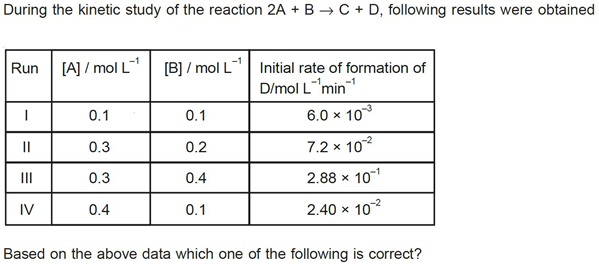(1) rate = k[A][B]2                     (2) rate = k[A]2[B]

(3) rate = k[A][B]                      (4) rate = k[A]2[B]2

61. For the reduction of silver ions with copper metal, the standard cell potential was found to be +0.46 V at 25°C. The value of standard Gibbs energy, ΔG0 will be (F = 96500 C mol–1)

(1) –98.0 kJ                               (2) –89.0 kJ

(3) –89.0 J                                 (4) –44.5 kJ

62. Which one of the following species does not exist under normal conditions?

(1) Li2                                        (2) Be2+

(3) Be2                                       (4) B2

63. AB crystallizes in a body centred cubic lattice with edge length ‘a’ equal to 387 pm. The distance between two oppositively charged ions in the lattice is

(1) 300 pm            (2) 335 pm

(3) 250 pm            (4) 200 pm

64. For an endothermic reaction, energy of activation is Ea and enthalpy of reaction is ΔH (both of these in kJ/mol). Minimum value of Ea will be

(1) Equal to zero              (2) Less than ΔH

(3) Equal to ΔH               (4) More than ΔH

65. Which one of the following ions has electronic configuration [Ar]3d6?

(1) Co3+                           (2) Ni3+

(3) Mn3+                          (4) Fe3+

66. In which of the following equilibrium Kc and KP are not equal?

(1)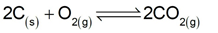(2)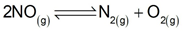(3)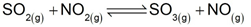(4)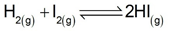67. If pH of a saturated solution of Ba(OH)2 is 12, the value of its K(SP) is

(1) 5.00 × 10–7 M3                     (2) 4.00 × 10–6 M3

(3) 4.00 × 10–7 M3                     (4) 5.00 × 10–6 M3

68. Which of the following ions will exhibit colour in aqueous solutions?

(1) Sc3+ (z = 21)              (2) La3+ (z = 57)

(3) Ti3+ (z = 22)               (4) Lu3+ (z = 71)

70. Which one of the following compounds is a peroxide?

(1) NO2                            (2) KO2

(3) BaO2                          (4) MnO2

71. In which of the following pairs of molecules/ions, the central atoms have sp2 hybridization?

(1) BF3 and NH2

(2) NO2 and NH3

(3) BF3 and NO2

(4) NH2 and H2O

72. The correct order of the decreasing ionic radii among the following isoelectronic species is

(1) K+ > Ca2+ > Cl > S2–

(2) Ca2+ > K+ > S2– > Cl

(3) Cl > S2– > Ca2+ > K+

(4) S2– > Cl > K+ > Ca2+

74. Which of the following complex ion is not expected to absorb visible light?

(1) [Ni(H2O6)]2+              (2) [Ni(CN)4]2–

(3) [Cr(NH3)6]3+              (4) [Fe(H2O)6]2+

75. Which of the following alkaline earth metal sulphates has hydration enthalpy higher than the lattice enthalpy?

(1) SrSO4                         (2) CaSO4

(3) BeSO4                        (4) BaSO4

76. The existence of two different coloured complexes with the composition of [Co(NH3)4Cl2]+ is due to

(1) Ionization isomerism            (2) Linkage isomerism

(3) Geometrical isomerism         (4) Cooridnation isomerism

77. Oxidation states of P in H4P2O5, H4P2O6, H4P2O7 are respectively

(1) + 3, + 4, + 5               (2) + 3, + 5, + 4

(3) + 5, + 3, + 4               (4) + 5, + 4, + 3